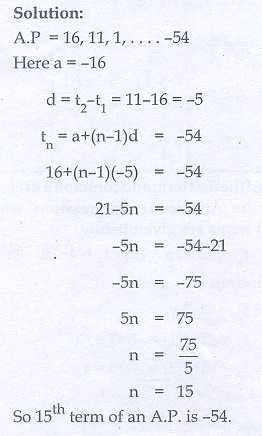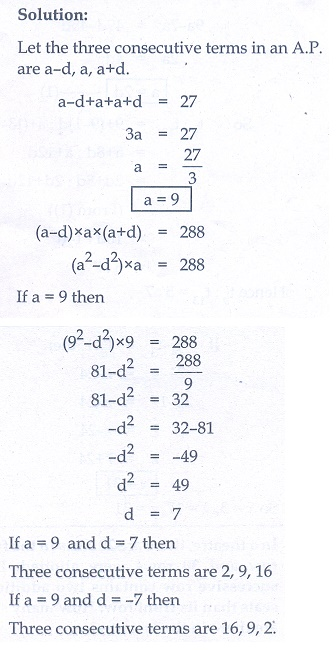Home | | Maths 10th Std | Exercise 2.5: Arithmetic Progression

# Exercise 2.5: Arithmetic Progression

Maths Book back answers and solution for Exercise questions - Mathematics : Numbers and Sequences: Arithmetic Progression: Exercise Questions with Answer

Exercise 2.5

1. Check whether the following sequences are in A.P.

(i) a - 3, a - 5, a - 7,...

(ii) ½, 1/3, ¼, 1/5, ….

(i) 9, 13, 17, 21, 25,...

(iv) -1/3 , 0, 1/3 , 2/3 ,...

(v) 1, - 1, 1, - 1, 1, -1,...2. First term a and common difference d are given below. Find the corresponding A.P.

(i) a = 5 , d = 6

(ii) a = 7 , d = −5

(iii) a = 3/4 , d = 1/23. Find the first term and common difference of the Arithmetic Progressions whose nth terms are given below

(i) tn  = −3 + 2n

(ii) tn  = 4 − 7n4. Find the 19th  term of an A.P. - 11, -15, -19,...5. Which term of an A.P. 16, 11, 6, 1,... is -54 ?6. Find the middle term(s) of an A.P. 9, 15, 21, 27,…,183.7. If nine times ninth term is equal to the fifteen times fifteenth term, show that six times twenty fourth term is zero.8. If 3 + k,  18 - k, 5k + 1 are in A.P. then find k.9. Find xy and z, given that the numbers x, 10, y, 24, z are in A.P.10. In a theatre, there are 20 seats in the front row and 30 rows were allotted. Each successive row contains two additional seats than its front row. How many seats are there in the last row?11. The sum of three consecutive terms that are in A.P. is 27 and their product is 288. Find the three terms.12. The ratio of 6th  and 8th  term of an A.P. is 7:9. Find the ratio of 9th  term to 13th  term.13. In a winter season let us take the temperature of Ooty from Monday to Friday to be in A.P. The sum of temperatures from Monday to Wednesday is 0° C and the sum of the temperatures from Wednesday to Friday is 18° C. Find the temperature on each of the five days.14. Priya earned ₹15,000 in the first month. Thereafter her salary increased by ₹1500 per year.  Her expenses are ₹13,000 during the first year and the expenses increases by ₹900 per year. How long will it take for her to save ₹20,000 per month.1.(i) A.P (ii) not an A.P (iii) A.P (iv) A.P (v) not an A.P

2.(i) 5, 11, 17, … (ii) 7, 2, -3 , … (iii) 3/4, 5/4, 7/4, ...

3.(i) -1 , 2 (ii) - -3 7 ,

4. -83

5. 15

6. 93, 99

8. 4

9. 3,17,31

10. 78

11. 2,9,16

12. 5:7

13. − °3 C, 0°C, 3°C, 6°C, 9°C

14. 31 years

Tags : Problem Questions with Answer, Solution | Mathematics , 10th Mathematics : UNIT 2 : Numbers and Sequences
Study Material, Lecturing Notes, Assignment, Reference, Wiki description explanation, brief detail
10th Mathematics : UNIT 2 : Numbers and Sequences : Exercise 2.5: Arithmetic Progression | Problem Questions with Answer, Solution | Mathematics